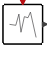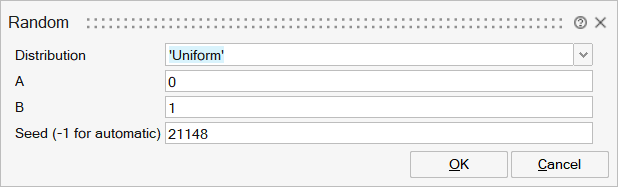# Random

This block is a random wave generator. Each output component takes piecewise constant random values. Each time an event is received on the input event port, the outputs take new independent random values.SignalGenerators

## Description

The Random block is a random value generator. Each output component takes piecewise constant random values. Each time an event is received on the input event port, the outputs take new independent random values.

Output port size is given by the size of A and B matrices. You can choose uniform distribution (default) or normal distribution.

In Uniform distribution, the range is defined as [A, A+B].

In Normal distribution A is mean and B is the deviation.

## ParametersNameLabelDescriptionData TypeValid Values

distribution

Distribution

Probability distribution. Default value: 'uniform'.

String

'Uniform'
'Normal'

A

A

First parameter for the distribution (lower value for uniform distribution, mean value for normal distribution). A real matrix, same size as matrix B. Default value: 0.

Matrix

B

B

Second parameter for the distribution (range value for uniform distribution, standard deviation for normal distribution). A real matrix, same size as matrix A. Default value: 1.

Matrix

seed

Seed (-1 for automatic)

Seed for the random generator (scalar). seed must be an integer between 0 and 2^32-1. Negative value for seed means automatic selection.

Scalar

## Ports

NameTypeDescriptionIO TypeNumber

Port 1

explicit

output

1

Port 2

activation

input

1

NameValueDescription

always active

no

Standard Mode is activated.

direct-feedthrough

no

zero-crossing

no

mode

no

continuous-time state

no

discrete-time state

yes